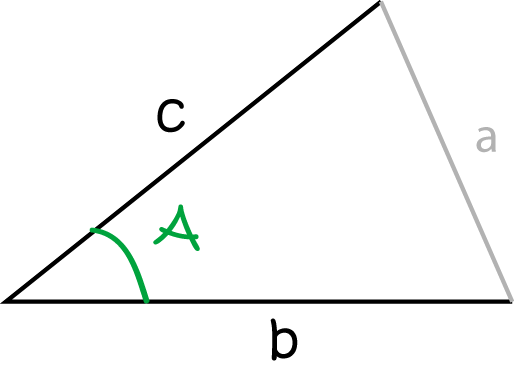# Definition of Included AngleAn included angle is the angle between two sides of a triangle.

The picture shows the angle $A$, which is the included angle between sides $b$ and $c$ of the triangle.

### Description

The aim of this dictionary is to provide definitions to common mathematical terms. Students learn a new math skill every week at school, sometimes just before they start a new skill, if they want to look at what a specific term means, this is where this dictionary will become handy and a go-to guide for a student.

### Audience

Year 1 to Year 12 students

### Learning Objectives

Learn common math terms starting with letter I

Author: Subject Coach
You must be logged in as Student to ask a Question.